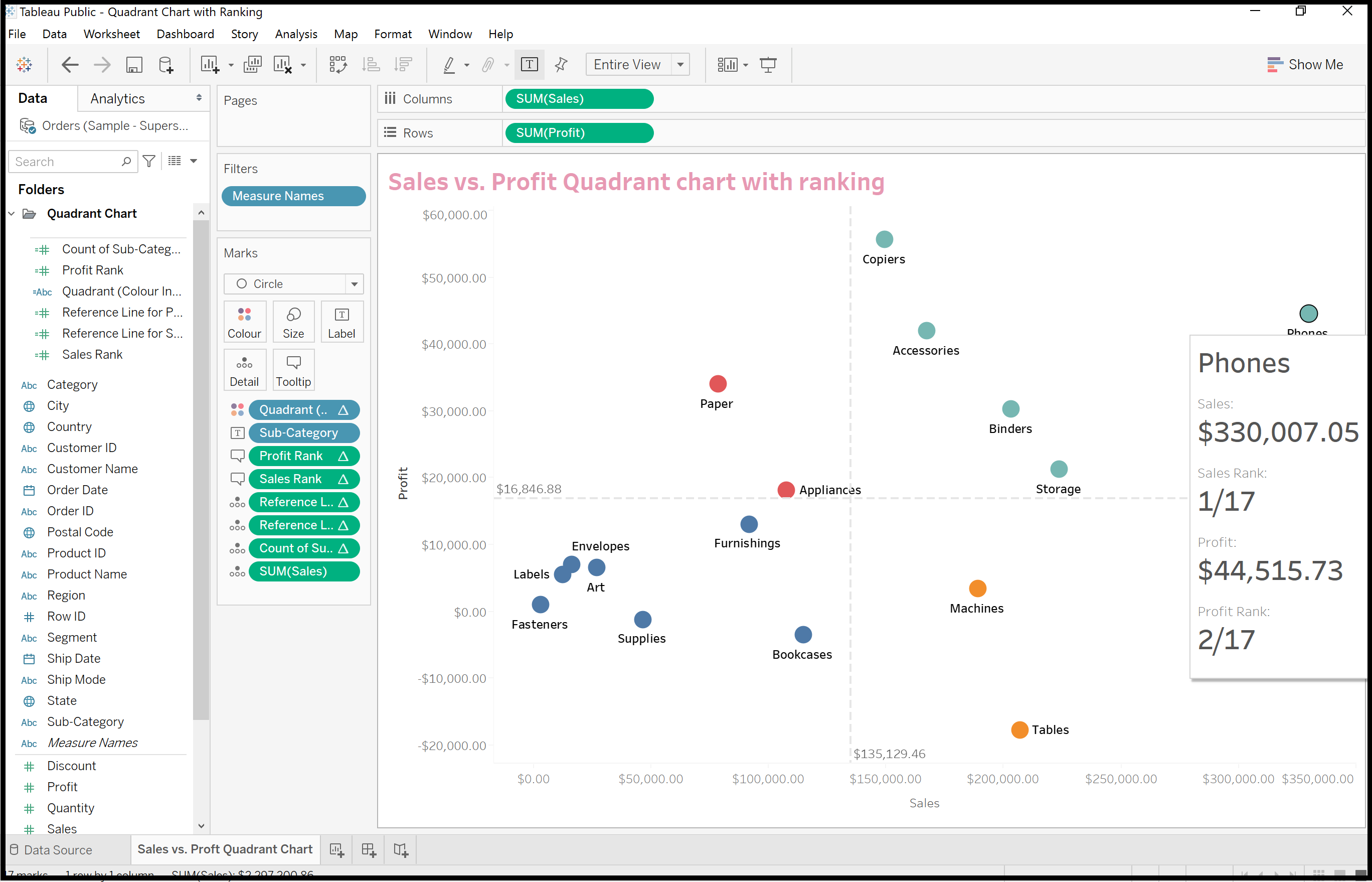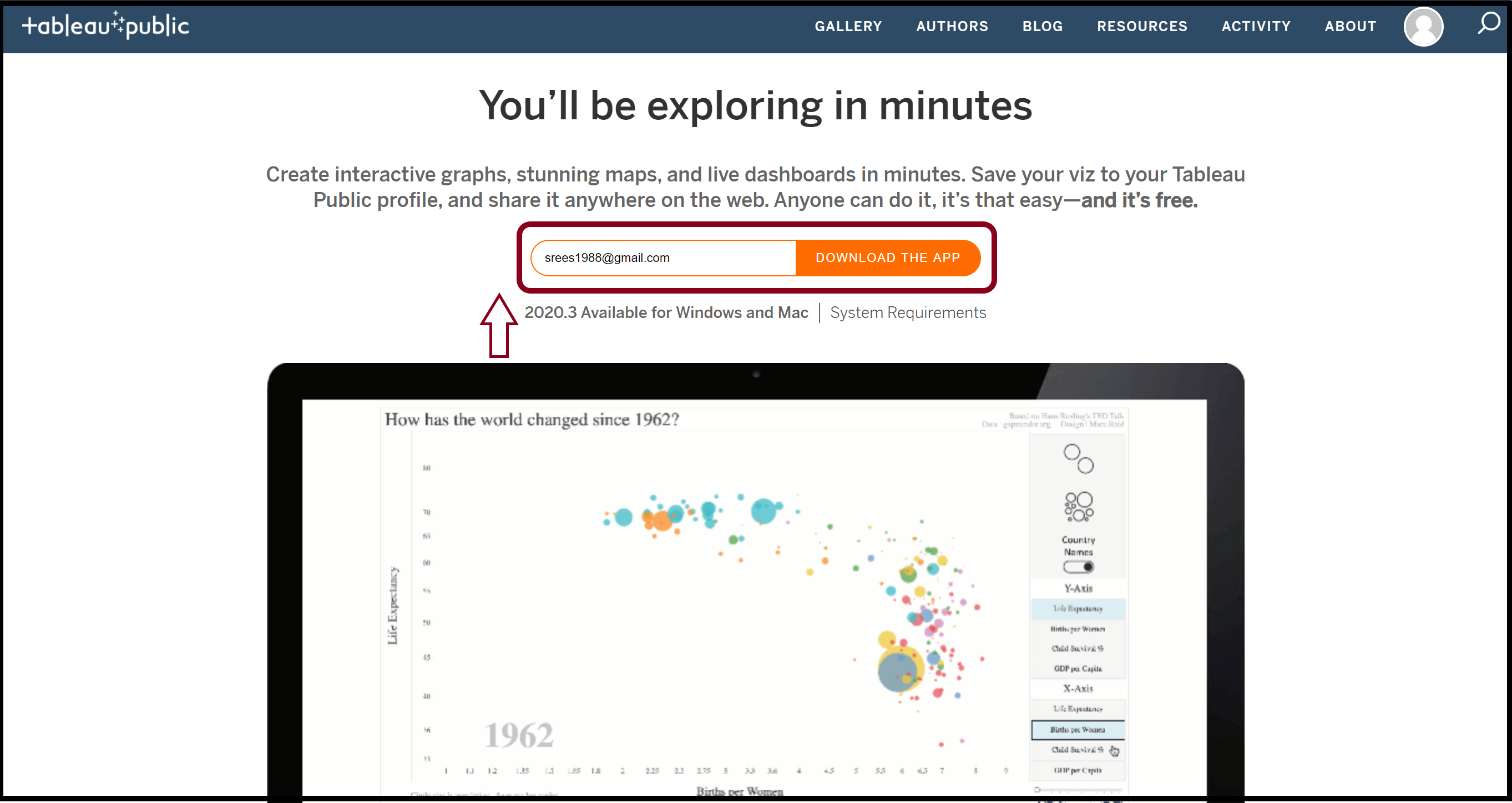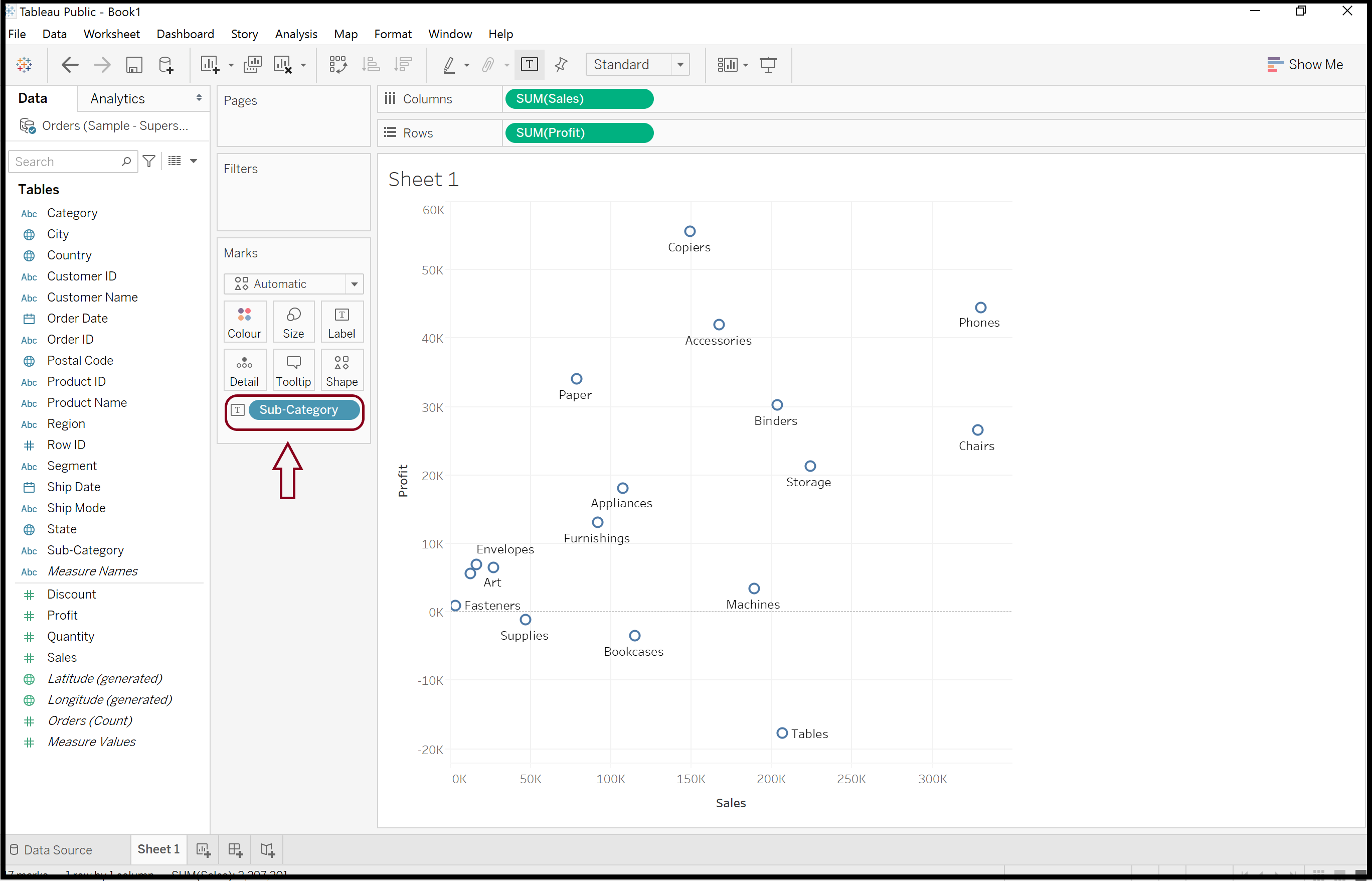Sree

Data Science & Analytics

# Quadrant Plots in Tableau

15 July 2020

### Objective

To quickly identify the best performing categories in any sales dataset using quadrant analysis in Tableau.### Details

A quadrant chart is nothing but a scatter plot that has four equal components. Each quadrant upholds data points that have similar characteristics. Here is a step-by-step approach on how to do a quadrant analysis plot in Tableau using the Superstore sales dataset so as to identify the best performing categories in terms of sales and profit:

##### Step 1:

Open a new Tableau file and import the ‘superstore’ public dataset into the workbook. In case if you haven’t worked with Tableau before, please download the ‘Tableau Public’ from the following URL: https://public.tableau.com/en-us/s/download##### Step 2:

Our objective is to evaluate the performance of various sub-categories based on sales and profit metrics. So, import ‘Orders’ table into the workspace; drag and drop aggregated ‘Sales’ and ‘Profit’ metrics into the rows & columns.

##### Step 3:

Bring in sub-categories to life by dragging and dropping the ‘sub-categories’ field to the ‘Label’ and the analytics pane.##### Step 4:

Create a calculated field for the reference lines:

``````Reference Line for Profit =
WINDOW_AVG(SUM([Profit]))

Reference Line for Sales =
WINDOW_AVG(SUM([Sales]))

``````

Drag both reference line calculations to ‘Detail’ and the analytics pane. Edit both reference line calculations, one for sales and the other for profit.

##### Step 5:

Create a calculated field for ‘Quadrant (Colour Indicator)’:

``````Quadrant (Colour Indicator)=

IF [Reference Line for Profit]>=
WINDOW_AVG(SUM([Profit]))
AND [Reference Line for Sales]>=
WINDOW_AVG(SUM([Sales]))
THEN 'UPPER RIGHT

ELSEIF

[Reference Line for Profit]<
WINDOW_AVG(SUM([Profit]))
AND [Reference Line for Sales]>=
WINDOW_AVG(SUM([Sales]))
THEN 'LOWER RIGHT'

ELSEIF

[Reference Line for Profit]>
WINDOW_AVG(SUM([Profit]))
AND [Reference Line for Sales]<
WINDOW_AVG(SUM([Sales]))
THEN 'UPPER LEFT'

ELSE 'LOWER LEFT'

END

``````

Drag ‘Quadrant (Colour Indicator’) to colour and edit the table calculation; select ‘sub-category’ as specific dimensions.

##### Step 6:

Further, create a calculated field for the rank and drag this to tooltip:

``````Sales Rank =
RANK(SUM([Sales]))

Profit Rank =
RANK(SUM([Profit]))

Count of Sub-Category =
WINDOW_COUNT(COUNTD([Sub-Category]))

``````

For each one of these calculated fields: Edit table calculation & select ‘sub-category’ as specific dimensions.

##### Step 7:

Give a title, format axes and add reference lines.

##### Step 8:

Fix the size of the BI widget and set up the tooltip.

``````<Sub-Category>
Sales:
<SUM(Sales)>

Sales Rank:
<AGG(Sales Rank)>/<AGG(Count of Sub-Category)>

Profit:
<SUM(Profit)>

Profit Rank:
<AGG(Profit Rank)>/<AGG(Count of Sub-Category)>

``````
##### Step 9: Generate Insights:(1) We can clearly see from the quadrant plots that phones, storage and binders get sold the most by yielding maximum sales and profit to the superstore. So, let the sales team know how important those products are for our annual revenue.

(2) On the other hand, we can see a large volume of tables and machines getting sold but their profit seems to be surprisingly lower than the peers. Are we underselling? Should we increase the price of the products?

(3) Not many Appliances are getting sold from the Superstore whilst their profit margin seems to be really high. Hence, let the sales team know that it would be good if the team can come up with more innovative measures to drive up the sales of those appliances.

(4) Lastly, revisit our sales and marketing strategy to ensure that an adequate number of furnishings, bookcases and art get sold with a revised markup price.

##### Conclusion

So, in this article, we briefly looked into the fundamentals of interactive quadrant analysis in Tableau and to reap insights from similar & dissimilar data points. From here, we can proceed with the exploratory data analysis either in R or Python to identify other significant components in the dataset that actually drive sales.

##### GitHub Repository

I have learned (and continue to learn) from many folks in Github. Hence sharing my Tableau file in a public GitHub Repository in case if it benefits any seekers online. Also, feel free to reach out to me if you need any help in understanding the fundamentals of Data visualization in Tableau. Happy to share what I know:) Hope this helps!